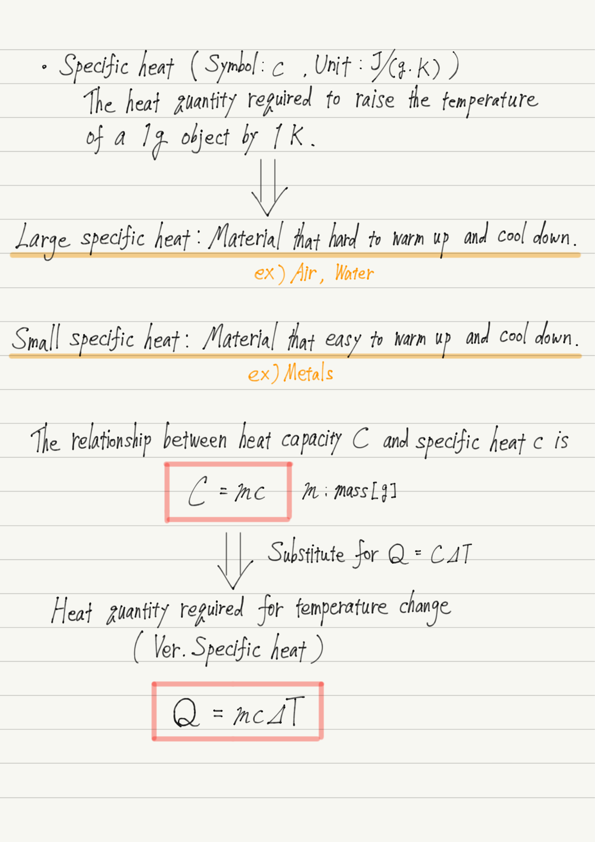# Specific Heat

Q. Which is harder to heat up, a lump of iron or water?

Is it water that is hard to warm up because metal warms up more easily?
Is it really so? In fact, this cannot be answered by itself because the question statement is insufficient.
What is insufficient is that the mass is not given.
For example, in this question, what if the iron is 1 kg and the water is 1 g?

When boiling water, the greater the volume of water, the harder it is to boil, as can be seen from the fact that the greater the volume, the harder it is to warm the same material.
No matter how easily a metal warms up, 1 kg of iron and 1 g of water will warm up more quickly than water.

But this comparison is not fair, is it?
If you want to compare which material is harder to heat up, you have to have the same mass to compare them.
This is where the concept of specific heat comes in.

## Definition of specific heat

The heat quantity required to raise the temperature of a 1 g object by 1 K is called specific heat.

Since the mass is set to 1 g, we can compare the specific heat of iron with that of water when comparing the resistance to warming as a material.

The specific heat of iron is about 0.45 J/(g・K) and that of water is about 4.2 J/(g・K), so it is true that water is less warm than iron when compared with iron of the same mass.

## Specific heat and Heat capacity

The specific heat of iron is 0.45 J/(g・K). What is the heat capacity of 1 kg of iron?

This is easy to understand if we consider the meaning of specific heat and heat capacity.
Specific heat : Heat quantity required to raise the temperature of a 1g object by 1K.
Heat capacity : Heat quantity required to raise the temperature of the entire object by 1K.

The specific heat of iron is 0.45 J/(g・K), which means that it takes 0.45 J to raise the temperature of 1 g of iron by 1 K.
Therefore, if you want to raise the temperature of 1 kg (=1000 g) of iron by 1 K, you will need 1000 times that amount of heat.

So the answer is 1000 g ✕ 0.45 J/(g・K) = 450 J/K.

Using this relationship between specific heat and heat capacity, we can create a specific heat version of the formula “Heat quantity required for temperature change” that we have worked on in the previous lecture.